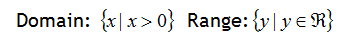Graphing
Example

Graph the function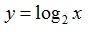Step 1. Switch the values of x and y and transform the log to exponential form.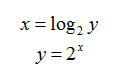Step 2. Make a table of values for the exponential function.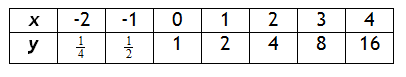Step 3. The table above is the inverse of the one needed so switch the input and output. This will create a set of ordered pairs that models the function.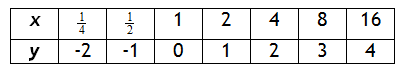Step 4. Graph the function.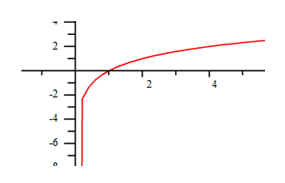Step 5. State the Domain & Range.

The x-values are approaching an asymptote at x = 0 and the y-values can be any number.